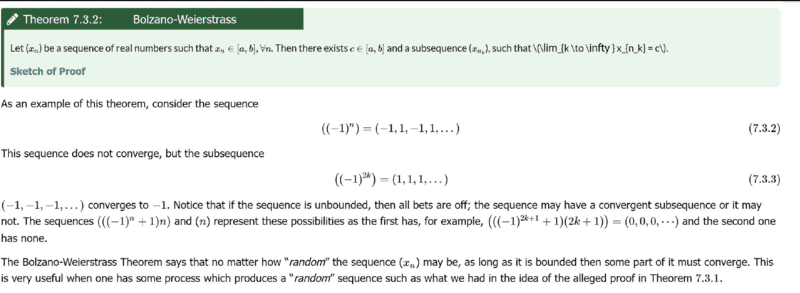# Understanding the Weierstrass- Bolzano theorem

• A
Gold Member
TL;DR Summary
I am currently looking at this theorem...basically it states that if you have a given sequence that is bounded and infinite then there exists atleast one limit point. Looking at the attached...i would like to know how the author concludes that the subsequence;

##{-1,-1,-1,...}## converges to ##-1##.

I guess that should follow from previous step...unless there is a mistake.Gold Member
Let me pick a sequence, say ##a_n=\dfrac{1}{n}## for example...this is a bounded infinite sequence. I can let my subsequence be defined by;

##b_n= \dfrac{1}{100},\dfrac{1}{200}, \dfrac{1}{300},...## the limit of this sequence will still tend to ##0##.

I am looking at it on the aspect of at least ##1## limit point. Question is, is there another limit point for the given sequence? using the given theorem?

•topsquark
Staff Emeritus
•